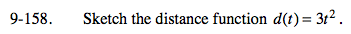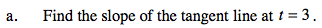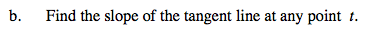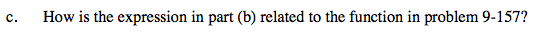### Home > PC > Chapter 9 > Lesson 9.3.5 > Problem9-158

9-158.$\lim_{ h \to 0} \frac{f(3+h)-f(3)}{3+h-3}=\lim_{ h \to 0} \frac{3(3+h)^2-3(3^2)}{h}=?$$\lim_{ h \to 0} \frac{f(t+h)-f(t)}{t+h-t}=\lim_{ h \to 0} \frac{3(t+h)^-3t^2}{h}=?$They are the same.